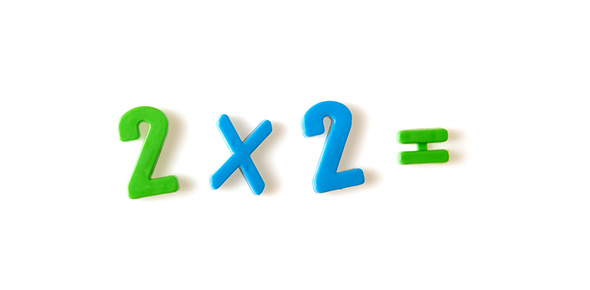# Multiplcation

10 Questions | Total Attempts: 28SettingsThis quiz is to test student knowledge of multiplication. It is designed for second graders.

• 1.
What is the product in a multiplication sentence?
• A.

The anwser

• B.

The numbers being multiplied

• C.

An array

• 2.
Jenny has four groups of five cookies. How many cookies does Jenny have in all?
• A.

4x4=20

• B.

4x5=20

• C.

4x5=25

• 3.
2x6=_____
• 4.
What is a factor in a multiplication sentence?
• A.

The numbers you multiply

• B.

The anwser

• C.

The signs

• 5.
What would be the repeated addition for 4x3?
• A.

4+4+4

• B.

3+3+3+3

• C.

2+2+2+2+2+2

• 6.
Which is the multiplication sentence for 6+6+6=18
• A.

2x6

• B.

3x6

• C.

2x3

• 7.
3x5=
• 8.
3x3=
• A.

12

• B.

6

• C.

9

• 9.
4+4+4=
• 10.
Write a multipication story problem for 2x5=10
Related Topics